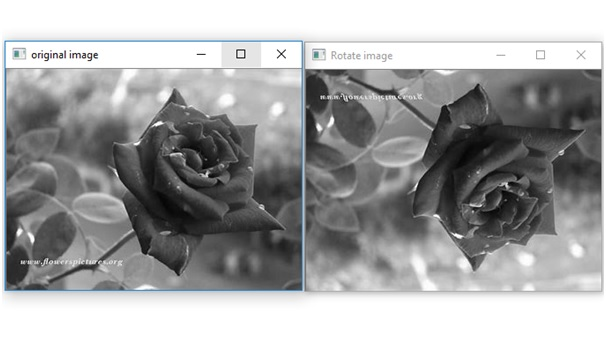# Rotate a grayscale image by 180 degree without using any inbuilt function in Python

In this article, we are going to learn how to rotate a grayscale image by 180 degree without using any inbuilt function in Python?
Submitted by Ankit Rai, on May 12, 2019

In this program, we will be using two functions of OpenCV-python (cv2) module.. let's see their syntax and descriptions first

It takes an absolute path/relative path of your image file as an argument and returns its corresponding image matrix.

If flag value is:

• 1: Loads a color image.
• 0: Loads image in grayscale mode.
• -1: Loads image as such including alpha channel.

If the flag value is not given then show the original image, which path is given.

2) imshow():
It takes window name and image matrix as an argument in order to display an image in a display window with a specified window name.

Also In this program, we will be using one attribute of an image matrix:

shape: This is the attribute of an image matrix which return shape of an image i.e. consisting of number of rows ,columns and number of planes.

In case of Gray Scale image, only one plane is present. If the number of planes is 1 than shape attribute only return number of rows and columns.

Also, in this program we are using the concept of array slicing

Let, A is 1-d array:
A[start:stop:step]

1. start: Starting number of the sequence.
2. stop: Generate numbers up to, but not including this number.
3. step: Difference between each number in the sequence.

Example:

```    A = [1,2,3,4,5,6,7,8,9,10]
print(A[ 10 : : -2])

Output:
[10, 8, 6,4,2]
```

### Python program to rotate a grayscale image by 180 degree without using any inbuilt functions

```# import numpy library as np
import numpy as np

# open-cv library is installed as cv2 in python
# import cv2 library into this program
import cv2

# we have to  pass the path of an image and flag value as 0

# displaying the image using imshow() function of cv2
# In this : 1st argument is name of the frame
# 2nd argument is the image matrix
cv2.imshow('original image',img)

# shape attribute of img matrix return tuple
# which contains no. of ros and columns present in img.
row,col = img.shape

# here we take all rows in reverse order and columns as before.
img = img[row-1: :-1, :]

# Show the image formed
cv2.imshow("Rotate image", img);
```

OutputPreparation